Publicité

# Industrial instrumentation flow measurement important equations

27 May 2021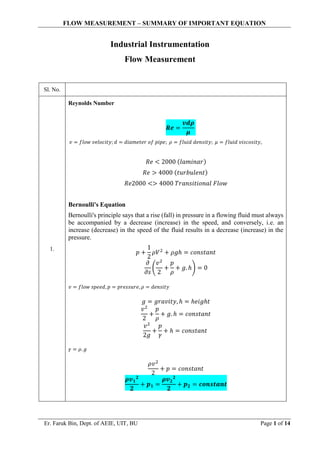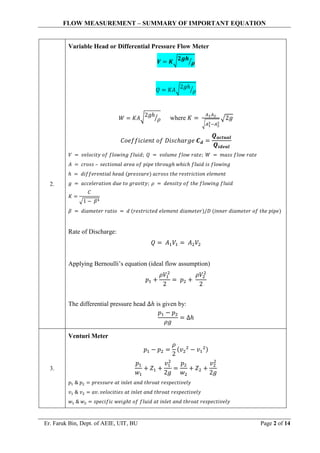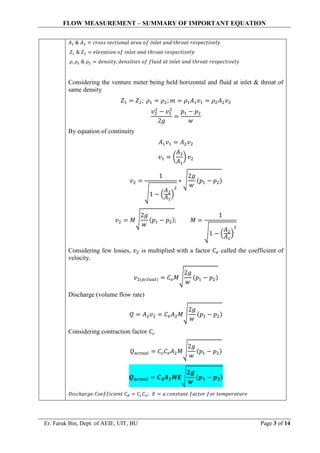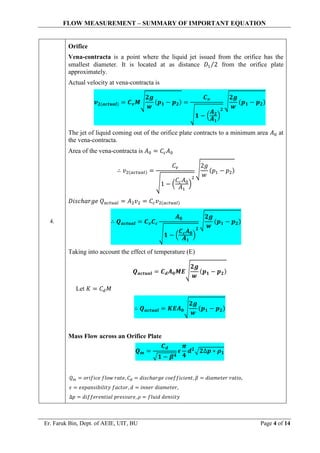Publicité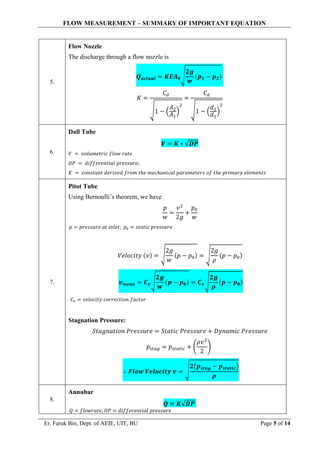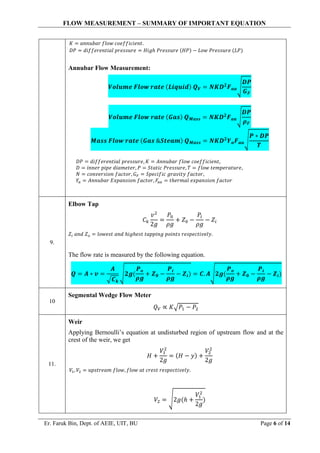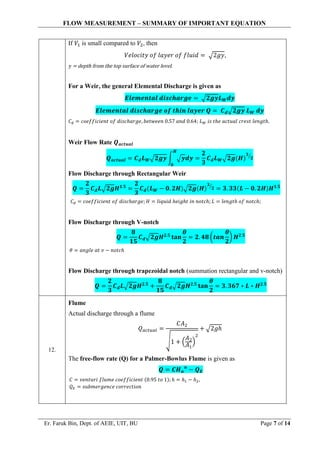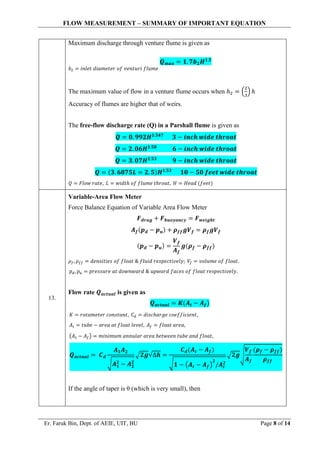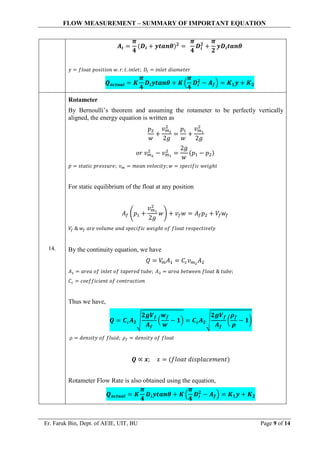Publicité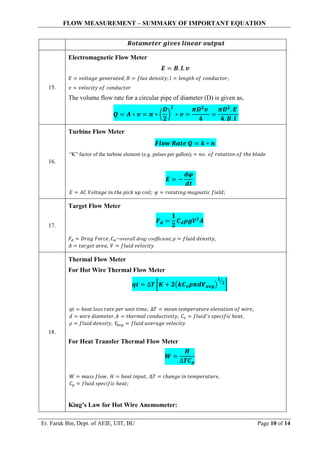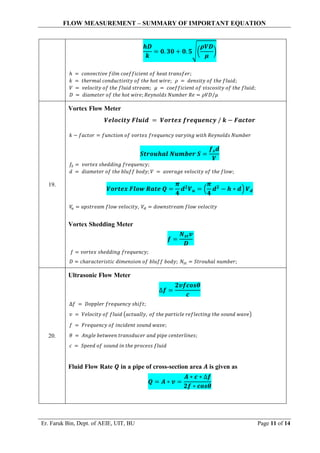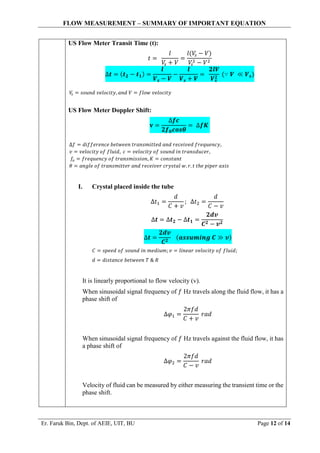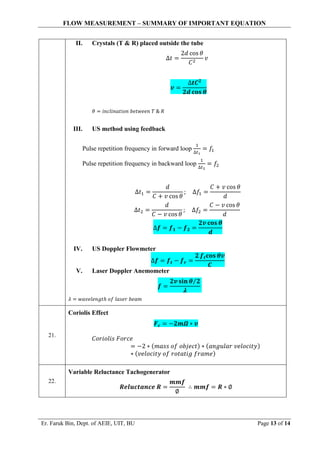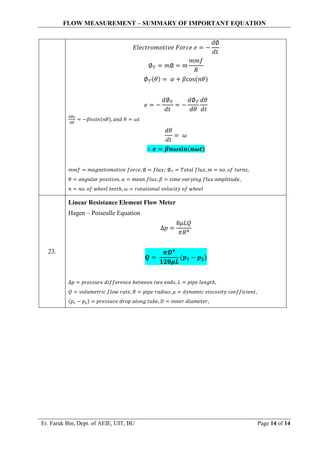Prochain SlideShare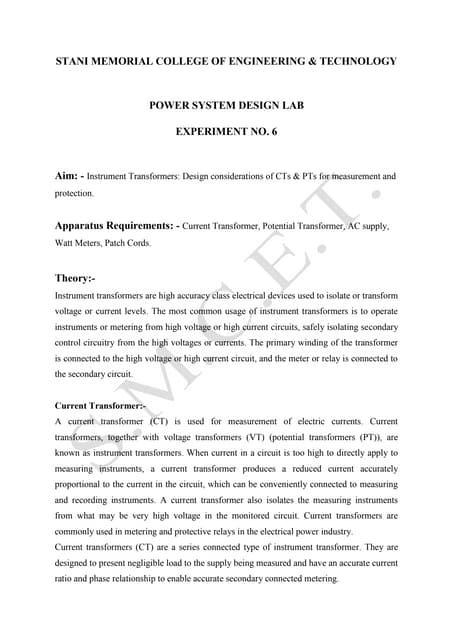Lab manual psd v sem experiment no 6
Chargement dans ... 3
1 sur 14
Publicité

### Industrial instrumentation flow measurement important equations

1. FLOW MEASUREMENT – SUMMARY OF IMPORTANT EQUATION Er. Faruk Bin, Dept. of AEIE, UIT, BU Page 1 of 14 Industrial Instrumentation Flow Measurement Sl. No. 1. Reynolds Number 𝑹𝒆 = 𝒗𝒅𝝆 𝝁 𝑣 = 𝑓𝑙𝑜𝑤 𝑣𝑒𝑙𝑜𝑐𝑖𝑡𝑦; 𝑑 = 𝑑𝑖𝑎𝑚𝑒𝑡𝑒𝑟 𝑜𝑓 𝑝𝑖𝑝𝑒; 𝜌 = 𝑓𝑙𝑢𝑖𝑑 𝑑𝑒𝑛𝑠𝑖𝑡𝑦; 𝜇 = 𝑓𝑙𝑢𝑖𝑑 𝑣𝑖𝑠𝑐𝑜𝑠𝑖𝑡𝑦, 𝑅𝑒 < 2000 (𝑙𝑎𝑚𝑖𝑛𝑎𝑟) 𝑅𝑒 > 4000 (𝑡𝑢𝑟𝑏𝑢𝑙𝑒𝑛𝑡) 𝑅𝑒2000 <> 4000 𝑇𝑟𝑎𝑛𝑠𝑖𝑡𝑖𝑜𝑛𝑎𝑙 𝐹𝑙𝑜𝑤 Bernoulli's Equation Bernoulli's principle says that a rise (fall) in pressure in a flowing fluid must always be accompanied by a decrease (increase) in the speed, and conversely, i.e. an increase (decrease) in the speed of the fluid results in a decrease (increase) in the pressure. 𝑝 + 1 2 𝜌𝑉2 + 𝜌𝑔ℎ = 𝑐𝑜𝑛𝑠𝑡𝑎𝑛𝑡 𝜕 𝜕𝑠 ( 𝑣2 2 + 𝑝 𝜌 + 𝑔. ℎ) = 0 𝑣 = 𝑓𝑙𝑜𝑤 𝑠𝑝𝑒𝑒𝑑, 𝑝 = 𝑝𝑟𝑒𝑠𝑠𝑢𝑟𝑒, 𝜌 = 𝑑𝑒𝑛𝑠𝑖𝑡𝑦 𝑔 = 𝑔𝑟𝑎𝑣𝑖𝑡𝑦, ℎ = ℎ𝑒𝑖𝑔ℎ𝑡 𝑣2 2 + 𝑝 𝜌 + 𝑔. ℎ = 𝑐𝑜𝑛𝑠𝑡𝑎𝑛𝑡 𝑣2 2𝑔 + 𝑝 𝛾 + ℎ = 𝑐𝑜𝑛𝑠𝑡𝑎𝑛𝑡 𝛾 = 𝜌. 𝑔 𝜌𝑣2 2 + 𝑝 = 𝑐𝑜𝑛𝑠𝑡𝑎𝑛𝑡 𝝆𝒗𝟏 𝟐 𝟐 + 𝒑𝟏 = 𝝆𝒗𝟐 𝟐 𝟐 + 𝒑𝟐 = 𝒄𝒐𝒏𝒔𝒕𝒂𝒏𝒕
2. FLOW MEASUREMENT – SUMMARY OF IMPORTANT EQUATION Er. Faruk Bin, Dept. of AEIE, UIT, BU Page 2 of 14 2. Variable Head or Differential Pressure Flow Meter 𝑽 = 𝑲√𝟐𝒈𝒉 𝝆 ⁄ 𝑄 = 𝐾𝐴√2𝑔ℎ 𝜌 ⁄ 𝑊 = 𝐾𝐴√2𝑔ℎ 𝜌 ⁄ where 𝐾 = 𝐴1𝐴2 √𝐴1 2−𝐴2 2 √2𝑔 𝐶𝑜𝑒𝑓𝑓𝑖𝑐𝑖𝑒𝑛𝑡 𝑜𝑓 𝐷𝑖𝑠𝑐ℎ𝑎𝑟𝑔𝑒 𝑪𝒅 = 𝑸𝒂𝒄𝒕𝒖𝒂𝒍 𝑸𝒊𝒅𝒆𝒂𝒍 𝑉 = 𝑣𝑒𝑙𝑜𝑐𝑖𝑡𝑦 𝑜𝑓 𝑓𝑙𝑜𝑤𝑖𝑛𝑔 𝑓𝑙𝑢𝑖𝑑; 𝑄 = 𝑣𝑜𝑙𝑢𝑚𝑒 𝑓𝑙𝑜𝑤 𝑟𝑎𝑡𝑒; 𝑊 = 𝑚𝑎𝑠𝑠 𝑓𝑙𝑜𝑤 𝑟𝑎𝑡𝑒 𝐴 = 𝑐𝑟𝑜𝑠𝑠 – 𝑠𝑒𝑐𝑡𝑖𝑜𝑛𝑎𝑙 𝑎𝑟𝑒𝑎 𝑜𝑓 𝑝𝑖𝑝𝑒 𝑡ℎ𝑟𝑜𝑢𝑔ℎ 𝑤ℎ𝑖𝑐ℎ 𝑓𝑙𝑢𝑖𝑑 𝑖𝑠 𝑓𝑙𝑜𝑤𝑖𝑛𝑔 ℎ = 𝑑𝑖𝑓𝑓𝑒𝑟𝑒𝑛𝑡𝑖𝑎𝑙 ℎ𝑒𝑎𝑑 (𝑝𝑟𝑒𝑠𝑠𝑢𝑟𝑒) 𝑎𝑐𝑟𝑜𝑠𝑠 𝑡ℎ𝑒 𝑟𝑒𝑠𝑡𝑟𝑖𝑐𝑡𝑖𝑜𝑛 𝑒𝑙𝑒𝑚𝑒𝑛𝑡 𝑔 = 𝑎𝑐𝑐𝑒𝑙𝑒𝑟𝑎𝑡𝑖𝑜𝑛 𝑑𝑢𝑒 𝑡𝑜 𝑔𝑟𝑎𝑣𝑖𝑡𝑦; 𝜌 = 𝑑𝑒𝑛𝑠𝑖𝑡𝑦 𝑜𝑓 𝑡ℎ𝑒 𝑓𝑙𝑜𝑤𝑖𝑛𝑔 𝑓𝑙𝑢𝑖𝑑 𝐾 = 𝐶 √1 − 𝛽4 𝛽 = 𝑑𝑖𝑎𝑚𝑒𝑡𝑒𝑟 𝑟𝑎𝑡𝑖𝑜 = 𝑑 (𝑟𝑒𝑠𝑡𝑟𝑖𝑐𝑡𝑒𝑑 𝑒𝑙𝑒𝑚𝑒𝑛𝑡 𝑑𝑖𝑎𝑚𝑒𝑡𝑒𝑟)/𝐷 (𝑖𝑛𝑛𝑒𝑟 𝑑𝑖𝑎𝑚𝑒𝑡𝑒𝑟 𝑜𝑓 𝑡ℎ𝑒 𝑝𝑖𝑝𝑒) Rate of Discharge: 𝑄 = 𝐴1𝑉1 = 𝐴2𝑉2 Applying Bernoulli’s equation (ideal flow assumption) 𝑝1 + 𝜌𝑉1 2 2 = 𝑝2 + 𝜌𝑉2 2 2 The differential pressure head ∆ℎ is given by: 𝑝1 − 𝑝2 𝜌𝑔 = ∆ℎ 3. Venturi Meter 𝑝1 − 𝑝2 = 𝜌 2 (𝑣2 2 − 𝑣1 2) 𝑝1 𝑤1 + 𝑍1 + 𝑣1 2 2𝑔 = 𝑝2 𝑤2 + 𝑍2 + 𝑣2 2 2𝑔 𝑝1 & 𝑝2 = 𝑝𝑟𝑒𝑠𝑠𝑢𝑟𝑒 𝑎𝑡 𝑖𝑛𝑙𝑒𝑡 𝑎𝑛𝑑 𝑡ℎ𝑟𝑜𝑎𝑡 𝑟𝑒𝑠𝑝𝑒𝑐𝑡𝑖𝑣𝑒𝑙𝑦 𝑣1 & 𝑣2 = 𝑎𝑣. 𝑣𝑒𝑙𝑜𝑐𝑖𝑡𝑖𝑒𝑠 𝑎𝑡 𝑖𝑛𝑙𝑒𝑡 𝑎𝑛𝑑 𝑡ℎ𝑟𝑜𝑎𝑡 𝑟𝑒𝑠𝑝𝑒𝑐𝑡𝑖𝑣𝑒𝑙𝑦 𝑤1 & 𝑤2 = 𝑠𝑝𝑒𝑐𝑖𝑓𝑖𝑐 𝑤𝑒𝑖𝑔ℎ𝑡 𝑜𝑓 𝑓𝑙𝑢𝑖𝑑 𝑎𝑡 𝑖𝑛𝑙𝑒𝑡 𝑎𝑛𝑑 𝑡ℎ𝑟𝑜𝑎𝑡 𝑟𝑒𝑠𝑝𝑒𝑐𝑡𝑖𝑣𝑒𝑙𝑦
3. FLOW MEASUREMENT – SUMMARY OF IMPORTANT EQUATION Er. Faruk Bin, Dept. of AEIE, UIT, BU Page 3 of 14 𝐴1 & 𝐴2 = 𝑐𝑟𝑜𝑠𝑠 𝑠𝑒𝑐𝑡𝑖𝑜𝑛𝑎𝑙 𝑎𝑟𝑒𝑎 𝑜𝑓 𝑖𝑛𝑙𝑒𝑡 𝑎𝑛𝑑 𝑡ℎ𝑟𝑜𝑎𝑡 𝑟𝑒𝑠𝑝𝑒𝑐𝑡𝑖𝑣𝑒𝑙𝑦 𝑍1 & 𝑍2 = 𝑒𝑙𝑒𝑣𝑎𝑡𝑖𝑜𝑛 𝑜𝑓 𝑖𝑛𝑙𝑒𝑡 𝑎𝑛𝑑 𝑡ℎ𝑟𝑜𝑎𝑡 𝑟𝑒𝑠𝑝𝑒𝑐𝑡𝑖𝑣𝑒𝑙𝑦 𝜌, 𝜌1 & 𝜌2 = 𝑑𝑒𝑛𝑠𝑖𝑡𝑦, 𝑑𝑒𝑛𝑠𝑖𝑡𝑖𝑒𝑠 𝑜𝑓 𝑓𝑙𝑢𝑖𝑑 𝑎𝑡 𝑖𝑛𝑙𝑒𝑡 𝑎𝑛𝑑 𝑡ℎ𝑟𝑜𝑎𝑡 𝑟𝑒𝑠𝑝𝑒𝑐𝑡𝑖𝑣𝑒𝑙𝑦 Considering the venture meter being held horizontal and fluid at inlet & throat of same density 𝑍1 = 𝑍2; 𝜌1 = 𝜌2; 𝑚 = 𝜌1𝐴1𝑣1 = 𝜌2𝐴2𝑣2 𝑣2 2 − 𝑣1 2 2𝑔 = 𝑝1 − 𝑝2 𝑤 By equation of continuity 𝐴1𝑣1 = 𝐴2𝑣2 𝑣1 = ( 𝐴2 𝐴1 ) 𝑣2 𝑣2 = 1 √1 − ( 𝐴2 𝐴1 ) 2 ∗ √ 2𝑔 𝑤 (𝑝1 − 𝑝2) 𝑣2 = 𝑀√ 2𝑔 𝑤 (𝑝1 − 𝑝2); 𝑀 = 1 √1 − ( 𝐴2 𝐴1 ) 2 Considering few losses, 𝑣2 is multiplied with a factor 𝐶𝑣 called the coefficient of velocity. 𝑣2(𝑎𝑐𝑡𝑢𝑎𝑙) = 𝐶𝑣𝑀√ 2𝑔 𝑤 (𝑝1 − 𝑝2) Discharge (volume flow rate) 𝑄 = 𝐴2𝑣2 = 𝐶𝑣𝐴2𝑀√ 2𝑔 𝑤 (𝑝1 − 𝑝2) Considering contraction factor 𝐶𝑐 𝑄𝑎𝑐𝑡𝑢𝑎𝑙 = 𝐶𝑐𝐶𝑣𝐴2𝑀√ 2𝑔 𝑤 (𝑝1 − 𝑝2) 𝑸𝒂𝒄𝒕𝒖𝒂𝒍 = 𝑪𝒅𝑨𝟐𝑴𝑬√ 𝟐𝒈 𝒘 (𝒑𝟏 − 𝒑𝟐) 𝐷𝑖𝑠𝑐ℎ𝑎𝑟𝑔𝑒 𝐶𝑜𝑒𝑓𝑓𝑖𝑐𝑖𝑒𝑛𝑡 𝐶𝑑 = 𝐶𝑐𝐶𝑣; 𝐸 = 𝑎 𝑐𝑜𝑛𝑠𝑡𝑎𝑛𝑡 𝑓𝑎𝑐𝑡𝑜𝑟 𝑓𝑜𝑟 𝑡𝑒𝑚𝑝𝑒𝑟𝑎𝑡𝑢𝑟𝑒
4. FLOW MEASUREMENT – SUMMARY OF IMPORTANT EQUATION Er. Faruk Bin, Dept. of AEIE, UIT, BU Page 4 of 14 4. Orifice Vena-contracta is a point where the liquid jet issued from the orifice has the smallest diameter. It is located at as distance 𝐷1 2 ⁄ from the orifice plate approximately. Actual velocity at vena-contracta is 𝒗𝟐(𝒂𝒄𝒕𝒖𝒂𝒍) = 𝑪𝒗𝑴√ 𝟐𝒈 𝒘 (𝒑𝟏 − 𝒑𝟐) = 𝑪𝒗 √𝟏 − ( 𝑨𝟐 𝑨𝟏 ) 𝟐 √ 𝟐𝒈 𝒘 (𝒑𝟏 − 𝒑𝟐) The jet of liquid coming out of the orifice plate contracts to a minimum area 𝐴0 at the vena-contracta. Area of the vena-contracta is 𝐴0 = 𝐶𝑐𝐴0 ∴ 𝑣2(𝑎𝑐𝑡𝑢𝑎𝑙) = 𝐶𝑣 √1 − ( 𝐶𝑐𝐴0 𝐴1 ) 2 √ 2𝑔 𝑤 (𝑝1 − 𝑝2) 𝐷𝑖𝑠𝑐ℎ𝑎𝑟𝑔𝑒 𝑄𝑎𝑐𝑡𝑢𝑎𝑙 = 𝐴2𝑣2 = 𝐶𝑐𝑣2(𝑎𝑐𝑡𝑢𝑎𝑙) ∴ 𝑸𝒂𝒄𝒕𝒖𝒂𝒍 = 𝑪𝒗𝑪𝒄 𝑨𝟎 √𝟏 − ( 𝑪𝒄𝑨𝟎 𝑨𝟏 ) 𝟐 √ 𝟐𝒈 𝒘 (𝒑𝟏 − 𝒑𝟐) Taking into account the effect of temperature (E) 𝑸𝒂𝒄𝒕𝒖𝒂𝒍 = 𝑪𝒅𝑨𝟎𝑴𝑬√ 𝟐𝒈 𝒘 (𝒑𝟏 − 𝒑𝟐) Let 𝐾 = 𝐶𝑑𝑀 ∴ 𝑸𝒂𝒄𝒕𝒖𝒂𝒍 = 𝑲𝑬𝑨𝟎√ 𝟐𝒈 𝒘 (𝒑𝟏 − 𝒑𝟐) Mass Flow across an Orifice Plate 𝑸𝒎 = 𝑪𝒅 √𝟏 − 𝜷𝟒 𝝐 𝝅 𝟒 𝒅𝟐 √𝟐∆𝒑 ∗ 𝝆𝟏 𝑄𝑚 = 𝑜𝑟𝑖𝑓𝑖𝑐𝑒 𝑓𝑙𝑜𝑤 𝑟𝑎𝑡𝑒, 𝐶𝑑 = 𝑑𝑖𝑠𝑐ℎ𝑎𝑟𝑔𝑒 𝑐𝑜𝑒𝑓𝑓𝑖𝑐𝑖𝑒𝑛𝑡, 𝛽 = 𝑑𝑖𝑎𝑚𝑒𝑡𝑒𝑟 𝑟𝑎𝑡𝑖𝑜, 𝜖 = 𝑒𝑥𝑝𝑎𝑛𝑠𝑖𝑏𝑖𝑙𝑖𝑡𝑦 𝑓𝑎𝑐𝑡𝑜𝑟, 𝑑 = 𝑖𝑛𝑛𝑒𝑟 𝑑𝑖𝑎𝑚𝑒𝑡𝑒𝑟, ∆𝑝 = 𝑑𝑖𝑓𝑓𝑒𝑟𝑒𝑛𝑡𝑖𝑎𝑙 𝑝𝑟𝑒𝑠𝑠𝑢𝑟𝑒, 𝜌 = 𝑓𝑙𝑢𝑖𝑑 𝑑𝑒𝑛𝑠𝑖𝑡𝑦
5. FLOW MEASUREMENT – SUMMARY OF IMPORTANT EQUATION Er. Faruk Bin, Dept. of AEIE, UIT, BU Page 5 of 14 5. Flow Nozzle The discharge through a flow nozzle is 𝑸𝒂𝒄𝒕𝒖𝒂𝒍 = 𝑲𝑬𝑨𝟎√ 𝟐𝒈 𝒘 (𝒑𝟏 − 𝒑𝟐) 𝐾 = 𝐶𝑑 √1 − ( 𝐴2 𝐴1 ) 2 = 𝐶𝑑 √1 − ( 𝑑2 𝑑1 ) 2 6. Dall Tube 𝑽 = 𝑲 ∗ √𝑫𝑷 𝑉 = 𝑣𝑜𝑙𝑢𝑚𝑒𝑡𝑟𝑖𝑐 𝑓𝑙𝑜𝑤 𝑟𝑎𝑡𝑒 𝐷𝑃 = 𝑑𝑖𝑓𝑓𝑒𝑟𝑒𝑛𝑡𝑖𝑎𝑙 𝑝𝑟𝑒𝑠𝑠𝑢𝑟𝑒; 𝐾 = 𝑐𝑜𝑛𝑠𝑡𝑎𝑛𝑡 𝑑𝑒𝑟𝑖𝑣𝑒𝑑 𝑓𝑟𝑜𝑚 𝑡ℎ𝑒 𝑚𝑒𝑐ℎ𝑎𝑛𝑖𝑐𝑎𝑙 𝑝𝑎𝑟𝑎𝑚𝑒𝑡𝑒𝑟𝑠 𝑜𝑓 𝑡ℎ𝑒 𝑝𝑟𝑖𝑚𝑎𝑟𝑦 𝑒𝑙𝑒𝑚𝑒𝑛𝑡𝑠 7. Pitot Tube Using Bernoulli’s theorem, we have 𝑝 𝑤 = 𝑣2 2𝑔 + 𝑝0 𝑤 𝑝 = 𝑝𝑟𝑒𝑠𝑠𝑢𝑟𝑒 𝑎𝑡 𝑖𝑛𝑙𝑒𝑡; 𝑝0 = 𝑠𝑡𝑎𝑡𝑖𝑐 𝑝𝑟𝑒𝑠𝑠𝑢𝑟𝑒 𝑉𝑒𝑙𝑜𝑐𝑖𝑡𝑦 (𝑣) = √ 2𝑔 𝑤 (𝑝 − 𝑝0) = √ 2𝑔 𝜌 (𝑝 − 𝑝0) 𝒗𝒎𝒆𝒂𝒏 = 𝑪𝒗√ 𝟐𝒈 𝒘 (𝒑 − 𝒑𝟎) = 𝑪𝒗√ 𝟐𝒈 𝝆 (𝒑 − 𝒑𝟎) 𝐶𝑣 = 𝑣𝑒𝑙𝑜𝑐𝑖𝑡𝑦 𝑐𝑜𝑟𝑟𝑒𝑐𝑡𝑖𝑜𝑛 𝑓𝑎𝑐𝑡𝑜𝑟 Stagnation Pressure: 𝑆𝑡𝑎𝑔𝑛𝑎𝑡𝑖𝑜𝑛 𝑃𝑟𝑒𝑠𝑠𝑢𝑟𝑒 = 𝑆𝑡𝑎𝑡𝑖𝑐 𝑃𝑟𝑒𝑠𝑠𝑢𝑟𝑒 + 𝐷𝑦𝑛𝑎𝑚𝑖𝑐 𝑃𝑟𝑒𝑠𝑠𝑢𝑟𝑒 𝑝𝑠𝑡𝑎𝑔 = 𝑝𝑠𝑡𝑎𝑡𝑖𝑐 + ( 𝜌𝑣2 2 ) ∴ 𝑭𝒍𝒐𝒘 𝑽𝒆𝒍𝒐𝒄𝒊𝒕𝒚 𝒗 = √ 𝟐(𝒑𝒔𝒕𝒂𝒈 − 𝒑𝒔𝒕𝒂𝒕𝒊𝒄) 𝝆 8. Annubar 𝑸 ∝ 𝑲√𝑫𝑷 𝑄 = 𝑓𝑙𝑜𝑤𝑟𝑎𝑡𝑒, 𝐷𝑃 = 𝑑𝑖𝑓𝑓𝑒𝑟𝑒𝑛𝑡𝑖𝑎𝑙 𝑝𝑟𝑒𝑠𝑠𝑢𝑟𝑒
6. FLOW MEASUREMENT – SUMMARY OF IMPORTANT EQUATION Er. Faruk Bin, Dept. of AEIE, UIT, BU Page 6 of 14 𝐾 = 𝑎𝑛𝑛𝑢𝑏𝑎𝑟 𝑓𝑙𝑜𝑤 𝑐𝑜𝑒𝑓𝑓𝑖𝑐𝑖𝑒𝑛𝑡. 𝐷𝑃 = 𝑑𝑖𝑓𝑓𝑒𝑟𝑒𝑛𝑡𝑖𝑎𝑙 𝑝𝑟𝑒𝑠𝑠𝑢𝑟𝑒 = 𝐻𝑖𝑔ℎ 𝑃𝑟𝑒𝑠𝑠𝑢𝑟𝑒 (𝐻𝑃) − 𝐿𝑜𝑤 𝑃𝑟𝑒𝑠𝑠𝑢𝑟𝑒 (𝐿𝑃) Annubar Flow Measurement: 𝑽𝒐𝒍𝒖𝒎𝒆 𝑭𝒍𝒐𝒘 𝒓𝒂𝒕𝒆 (𝑳𝒊𝒒𝒖𝒊𝒅) 𝑸𝑽 = 𝑵𝑲𝑫𝟐 𝑭𝒂𝒂√ 𝑫𝑷 𝑮𝑭 𝑽𝒐𝒍𝒖𝒎𝒆 𝑭𝒍𝒐𝒘 𝒓𝒂𝒕𝒆 (𝑮𝒂𝒔) 𝑸𝑴𝒂𝒔𝒔 = 𝑵𝑲𝑫𝟐 𝑭𝒂𝒂√ 𝑫𝑷 𝝆𝑭 𝑴𝒂𝒔𝒔 𝑭𝒍𝒐𝒘 𝒓𝒂𝒕𝒆 (𝑮𝒂𝒔 &𝑺𝒕𝒆𝒂𝒎) 𝑸𝑴𝒂𝒔𝒔 = 𝑵𝑲𝑫𝟐 𝒀𝒂𝑭𝒂𝒂√ 𝑷 ∗ 𝑫𝑷 𝑻 𝐷𝑃 = 𝑑𝑖𝑓𝑓𝑒𝑟𝑒𝑛𝑡𝑖𝑎𝑙 𝑝𝑟𝑒𝑠𝑠𝑢𝑟𝑒, 𝐾 = 𝐴𝑛𝑛𝑢𝑏𝑎𝑟 𝑓𝑙𝑜𝑤 𝑐𝑜𝑒𝑓𝑓𝑖𝑐𝑖𝑒𝑛𝑡, 𝐷 = 𝑖𝑛𝑛𝑒𝑟 𝑝𝑖𝑝𝑒 𝑑𝑖𝑎𝑚𝑒𝑡𝑒𝑟, 𝑃 = 𝑆𝑡𝑎𝑡𝑖𝑐 𝑃𝑟𝑒𝑠𝑠𝑢𝑟𝑒, 𝑇 = 𝑓𝑙𝑜𝑤 𝑡𝑒𝑚𝑝𝑒𝑟𝑎𝑡𝑢𝑟𝑒, 𝑁 = 𝑐𝑜𝑛𝑣𝑒𝑟𝑠𝑖𝑜𝑛 𝑓𝑎𝑐𝑡𝑜𝑟, 𝐺𝐹 = 𝑆𝑝𝑒𝑐𝑖𝑓𝑖𝑐 𝑔𝑟𝑎𝑣𝑖𝑡𝑦 𝑓𝑎𝑐𝑡𝑜𝑟, 𝑌𝑎 = 𝐴𝑛𝑛𝑢𝑏𝑎𝑟 𝐸𝑥𝑝𝑎𝑛𝑠𝑖𝑜𝑛 𝑓𝑎𝑐𝑡𝑜𝑟, 𝐹𝑎𝑎 = 𝑡ℎ𝑒𝑟𝑚𝑎𝑙 𝑒𝑥𝑝𝑎𝑛𝑠𝑖𝑜𝑛 𝑓𝑎𝑐𝑡𝑜𝑟 9. Elbow Tap 𝐶𝑘 𝑣2 2𝑔 = 𝑃0 𝜌𝑔 + 𝑍0 − 𝑃𝑖 𝜌𝑔 − 𝑍𝑖 𝑍𝑖 𝑎𝑛𝑑 𝑍𝑜 = 𝑙𝑜𝑤𝑒𝑠𝑡 𝑎𝑛𝑑 ℎ𝑖𝑔ℎ𝑒𝑠𝑡 𝑡𝑎𝑝𝑝𝑖𝑛𝑔 𝑝𝑜𝑖𝑛𝑡𝑠 𝑟𝑒𝑠𝑝𝑒𝑐𝑡𝑖𝑣𝑒𝑙𝑦. The flow rate is measured by the following equation. 𝑸 = 𝑨 ∗ 𝒗 = 𝑨 √𝑪𝒌 √𝟐𝒈( 𝑷𝒐 𝝆𝒈 + 𝒁𝟎 − 𝑷𝒊 𝝆𝒈 − 𝒁𝒊) = 𝑪. 𝑨√𝟐𝒈( 𝑷𝒐 𝝆𝒈 + 𝒁𝟎 − 𝑷𝒊 𝝆𝒈 − 𝒁𝒊) 10 Segmental Wedge Flow Meter 𝑄𝑉 ∝ 𝐾√𝑃1 − 𝑃2 11. Weir Applying Bernoulli’s equation at undisturbed region of upstream flow and at the crest of the weir, we get 𝐻 + 𝑉1 2 2𝑔 = (𝐻 − 𝑦) + 𝑉2 2 2𝑔 𝑉1, 𝑉2 = 𝑢𝑝𝑠𝑡𝑟𝑒𝑎𝑚 𝑓𝑙𝑜𝑤, 𝑓𝑙𝑜𝑤 𝑎𝑡 𝑐𝑟𝑒𝑠𝑡 𝑟𝑒𝑠𝑝𝑒𝑐𝑡𝑖𝑣𝑒𝑙𝑦. 𝑉2 = √2𝑔(ℎ + 𝑉1 2 2𝑔 )
7. FLOW MEASUREMENT – SUMMARY OF IMPORTANT EQUATION Er. Faruk Bin, Dept. of AEIE, UIT, BU Page 7 of 14 If 𝑉1 is small compared to 𝑉2, then 𝑉𝑒𝑙𝑜𝑐𝑖𝑡𝑦 𝑜𝑓 𝑙𝑎𝑦𝑒𝑟 𝑜𝑓 𝑓𝑙𝑢𝑖𝑑 = √2𝑔𝑦, 𝑦 = depth from the top surface of water level. For a Weir, the general Elemental Discharge is given as 𝑬𝒍𝒆𝒎𝒆𝒏𝒕𝒂𝒍 𝒅𝒊𝒔𝒄𝒉𝒂𝒓𝒈𝒆 = √𝟐𝒈𝒚𝑳𝑾𝒅𝒚 𝑬𝒍𝒆𝒎𝒆𝒏𝒕𝒂𝒍 𝒅𝒊𝒔𝒄𝒉𝒂𝒓𝒈𝒆 𝒐𝒇 𝒕𝒉𝒊𝒏 𝒍𝒂𝒚𝒆𝒓 𝑸 = 𝑪𝒅√𝟐𝒈𝒚 𝑳𝑾 𝒅𝒚 𝐶𝑑 = 𝑐𝑜𝑒𝑓𝑓𝑖𝑐𝑖𝑒𝑛𝑡 𝑜𝑓 𝑑𝑖𝑠𝑐ℎ𝑎𝑟𝑔𝑒, 𝑏𝑒𝑡𝑤𝑒𝑒𝑛 0.57 𝑎𝑛𝑑 0.64; 𝐿𝑊 𝑖𝑠 𝑡ℎ𝑒 𝑎𝑐𝑡𝑢𝑎𝑙 𝑐𝑟𝑒𝑠𝑡 𝑙𝑒𝑛𝑔𝑡ℎ. Weir Flow Rate 𝑸𝒂𝒄𝒕𝒖𝒂𝒍 𝑸𝒂𝒄𝒕𝒖𝒂𝒍 = 𝑪𝒅𝑳𝑾√𝟐𝒈𝒚 ∫ √𝒚𝒅𝒚 𝑯 𝟎 = 𝟐 𝟑 𝑪𝒅𝑳𝑾√𝟐𝒈(𝑯) 𝟑 𝟐 ⁄ Flow Discharge through Rectangular Weir 𝑸 = 𝟐 𝟑 𝑪𝒅𝑳√𝟐𝒈𝑯𝟏.𝟓 = 𝟐 𝟑 𝑪𝒅(𝑳𝑾 − 𝟎. 𝟐𝑯)√𝟐𝒈(𝑯) 𝟑 𝟐 ⁄ = 𝟑. 𝟑𝟑(𝑳 − 𝟎. 𝟐𝑯)𝑯𝟏.𝟓 𝐶𝑑 = 𝑐𝑜𝑒𝑓𝑓𝑖𝑐𝑖𝑒𝑛𝑡 𝑜𝑓 𝑑𝑖𝑠𝑐ℎ𝑎𝑟𝑔𝑒; 𝐻 = 𝑙𝑖𝑞𝑢𝑖𝑑 ℎ𝑒𝑖𝑔ℎ𝑡 𝑖𝑛 𝑛𝑜𝑡𝑐ℎ; 𝐿 = 𝑙𝑒𝑛𝑔𝑡ℎ 𝑜𝑓 𝑛𝑜𝑡𝑐ℎ; Flow Discharge through V-notch 𝑸 = 𝟖 𝟏𝟓 𝑪𝒅√𝟐𝒈𝑯𝟐.𝟓 𝐭𝐚𝐧 𝜽 𝟐 = 𝟐. 𝟒𝟖 (𝒕𝒂𝒏 𝜽 𝟐 ) 𝑯𝟐.𝟓 𝜃 = 𝑎𝑛𝑔𝑙𝑒 𝑎𝑡 𝑣 − 𝑛𝑜𝑡𝑐ℎ Flow Discharge through trapezoidal notch (summation rectangular and v-notch) 𝑸 = 𝟐 𝟑 𝑪𝒅𝑳√𝟐𝒈𝑯𝟐.𝟓 + 𝟖 𝟏𝟓 𝑪𝒅√𝟐𝒈𝑯𝟐.𝟓 𝐭𝐚𝐧 𝜽 𝟐 = 𝟑. 𝟑𝟔𝟕 ∗ 𝑳 ∗ 𝑯𝟐.𝟓 12. Flume Actual discharge through a flume 𝑄𝑎𝑐𝑡𝑢𝑎𝑙 = 𝐶𝐴2 √1 + ( 𝐴2 𝐴1 ) 2 + √2𝑔ℎ The free-flow rate (Q) for a Palmer-Bowlus Flume is given as 𝑸 = 𝑪𝑯𝒂 𝒏 − 𝑸𝑬 𝐶 = 𝑣𝑒𝑛𝑡𝑢𝑟𝑖 𝑓𝑙𝑢𝑚𝑒 𝑐𝑜𝑒𝑓𝑓𝑖𝑐𝑖𝑒𝑛𝑡 (0.95 𝑡𝑜 1); ℎ = ℎ1 − ℎ2, 𝑄𝐸 = 𝑠𝑢𝑏𝑚𝑒𝑟𝑔𝑒𝑛𝑐𝑒 𝑐𝑜𝑟𝑟𝑒𝑐𝑡𝑖𝑜𝑛
8. FLOW MEASUREMENT – SUMMARY OF IMPORTANT EQUATION Er. Faruk Bin, Dept. of AEIE, UIT, BU Page 8 of 14 Maximum discharge through venture flume is given as 𝑸𝒎𝒂𝒙 = 𝟏. 𝟕𝒃𝟐𝑯𝟏.𝟓 𝑏2 = 𝑖𝑛𝑙𝑒𝑡 𝑑𝑖𝑎𝑚𝑒𝑡𝑒𝑟 𝑜𝑓 𝑣𝑒𝑛𝑡𝑢𝑟𝑖 𝑓𝑙𝑢𝑚𝑒 The maximum value of flow in a venture flume occurs when ℎ2 = ( 2 3 ) ℎ Accuracy of flumes are higher that of weirs. The free-flow discharge rate (Q) in a Parshall flume is given as 𝑸 = 𝟎. 𝟗𝟗𝟐𝑯𝟏.𝟓𝟒𝟕 𝟑 − 𝒊𝒏𝒄𝒉 𝒘𝒊𝒅𝒆 𝒕𝒉𝒓𝒐𝒂𝒕 𝑸 = 𝟐. 𝟎𝟔𝑯𝟏.𝟓𝟖 𝟔 − 𝒊𝒏𝒄𝒉 𝒘𝒊𝒅𝒆 𝒕𝒉𝒓𝒐𝒂𝒕 𝑸 = 𝟑. 𝟎𝟕𝑯𝟏.𝟓𝟑 𝟗 − 𝒊𝒏𝒄𝒉 𝒘𝒊𝒅𝒆 𝒕𝒉𝒓𝒐𝒂𝒕 𝑸 = (𝟑. 𝟔𝟖𝟕𝟓𝑳 = 𝟐. 𝟓)𝑯𝟏.𝟓𝟑 𝟏𝟎 − 𝟓𝟎 𝒇𝒆𝒆𝒕 𝒘𝒊𝒅𝒆 𝒕𝒉𝒓𝒐𝒂𝒕 𝑄 = 𝐹𝑙𝑜𝑤 𝑟𝑎𝑡𝑒, 𝐿 = 𝑤𝑖𝑑𝑡ℎ 𝑜𝑓 𝑓𝑙𝑢𝑚𝑒 𝑡ℎ𝑟𝑜𝑎𝑡, 𝐻 = 𝐻𝑒𝑎𝑑 (𝑓𝑒𝑒𝑡) 13. Variable-Area Flow Meter Force Balance Equation of Variable Area Flow Meter 𝑭𝒅𝒓𝒂𝒈 + 𝑭𝒃𝒖𝒐𝒚𝒂𝒏𝒄𝒚 = 𝑭𝒘𝒆𝒊𝒈𝒉𝒕 𝑨𝒇(𝒑𝒅 − 𝒑𝒖) + 𝝆𝒇𝒇𝒈𝑽𝒇 = 𝝆𝒇𝒈𝑽𝒇 (𝒑𝒅 − 𝒑𝒖) = 𝑽𝒇 𝑨𝒇 𝒈(𝝆𝒇 − 𝝆𝒇𝒇) 𝜌𝑓, 𝜌𝑓𝑓 = 𝑑𝑒𝑛𝑠𝑖𝑡𝑖𝑒𝑠 𝑜𝑓 𝑓𝑙𝑜𝑎𝑡 & 𝑓𝑙𝑢𝑖𝑑 𝑟𝑒𝑠𝑝𝑒𝑐𝑡𝑖𝑣𝑒𝑙𝑦; 𝑉𝑓 = 𝑣𝑜𝑙𝑢𝑚𝑒 𝑜𝑓 𝑓𝑙𝑜𝑎𝑡. 𝑝𝑑, 𝑝𝑢 = 𝑝𝑟𝑒𝑠𝑠𝑢𝑟𝑒 𝑎𝑡 𝑑𝑜𝑤𝑛𝑤𝑎𝑟𝑑 & 𝑢𝑝𝑤𝑎𝑟𝑑 𝑓𝑎𝑐𝑒𝑠 𝑜𝑓 𝑓𝑙𝑜𝑎𝑡 𝑟𝑒𝑠𝑝𝑒𝑐𝑡𝑖𝑣𝑒𝑙𝑦. Flow rate 𝑸𝒂𝒄𝒕𝒖𝒂𝒍 is given as 𝑸𝒂𝒄𝒕𝒖𝒂𝒍 = 𝑲(𝑨𝒕 − 𝑨𝒇) 𝐾 = 𝑟𝑜𝑡𝑎𝑚𝑒𝑡𝑒𝑟 𝑐𝑜𝑛𝑠𝑡𝑎𝑛𝑡, 𝐶𝑑 = 𝑑𝑖𝑠𝑐ℎ𝑎𝑟𝑔𝑒 𝑐𝑜𝑒𝑓𝑓𝑖𝑐𝑖𝑒𝑛𝑡, 𝐴𝑡 = 𝑡𝑢𝑏𝑒 − 𝑎𝑟𝑒𝑎 𝑎𝑡 𝑓𝑙𝑜𝑎𝑡 𝑙𝑒𝑣𝑒𝑙, 𝐴𝑓 = 𝑓𝑙𝑜𝑎𝑡 𝑎𝑟𝑒𝑎, (𝐴𝑡 − 𝐴𝑓) = 𝑚𝑖𝑛𝑖𝑚𝑢𝑚 𝑎𝑛𝑛𝑢𝑙𝑎𝑟 𝑎𝑟𝑒𝑎 𝑏𝑒𝑡𝑤𝑒𝑒𝑛 𝑡𝑢𝑏𝑒 𝑎𝑛𝑑 𝑓𝑙𝑜𝑎𝑡, 𝑸𝒂𝒄𝒕𝒖𝒂𝒍 = 𝑪𝒅 𝑨𝟏𝑨𝟐 √𝑨𝟏 𝟐 − 𝑨𝟐 𝟐 √𝟐𝒈√∆𝒉 = 𝑪𝒅(𝑨𝒕 − 𝑨𝒇) √𝟏 − (𝑨𝒕 − 𝑨𝒇) 𝟐 /𝑨𝒕 𝟐 √𝟐𝒈√ 𝑽𝒇 𝑨𝒇 (𝝆𝒇 − 𝝆𝒇𝒇) 𝝆𝒇𝒇 If the angle of taper is θ (which is very small), then
9. FLOW MEASUREMENT – SUMMARY OF IMPORTANT EQUATION Er. Faruk Bin, Dept. of AEIE, UIT, BU Page 9 of 14 𝑨𝒕 = 𝝅 𝟒 (𝑫𝒊 + 𝒚𝒕𝒂𝒏𝜽)𝟐 = 𝝅 𝟒 𝑫𝒊 𝟐 + 𝝅 𝟐 𝒚𝑫𝒊𝒕𝒂𝒏𝜽 𝑦 = 𝑓𝑙𝑜𝑎𝑡 𝑝𝑜𝑠𝑖𝑡𝑖𝑜𝑛 𝑤. 𝑟. 𝑡. 𝑖𝑛𝑙𝑒𝑡; 𝐷𝑖 = 𝑖𝑛𝑙𝑒𝑡 𝑑𝑖𝑎𝑚𝑒𝑡𝑒𝑟 𝑸𝒂𝒄𝒕𝒖𝒂𝒍 = 𝑲 𝝅 𝟒 𝑫𝒊𝒚𝒕𝒂𝒏𝜽 + 𝑲 ( 𝝅 𝟒 𝑫𝒊 𝟐 − 𝑨𝒇) = 𝑲𝟏𝒚 + 𝑲𝟐 14. Rotameter By Bernoulli’s theorem and assuming the rotameter to be perfectly vertically aligned, the energy equation is written as 𝑝2 𝑤 + 𝑣𝑚2 2 2𝑔 = 𝑝1 𝑤 + 𝑣𝑚1 2 2𝑔 𝑜𝑟 𝑣𝑚2 2 − 𝑣𝑚1 2 = 2𝑔 𝑤 (𝑝1 − 𝑝2) 𝑝 = 𝑠𝑡𝑎𝑡𝑖𝑐 𝑝𝑟𝑒𝑠𝑠𝑢𝑟𝑒; 𝑣𝑚 = 𝑚𝑒𝑎𝑛 𝑣𝑒𝑙𝑜𝑐𝑖𝑡𝑦; 𝑤 = 𝑠𝑝𝑒𝑐𝑖𝑓𝑖𝑐 𝑤𝑒𝑖𝑔ℎ𝑡 For static equilibrium of the float at any position 𝐴𝑓 (𝑝1 + 𝑣𝑚1 2 2𝑔 𝑤) + 𝑣𝑓𝑤 = 𝐴𝑓𝑝2 + 𝑉𝑓𝑤𝑓 𝑉𝑓 & 𝑤𝑓 𝑎𝑟𝑒 𝑣𝑜𝑙𝑢𝑚𝑒 𝑎𝑛𝑑 𝑠𝑝𝑒𝑐𝑖𝑓𝑖𝑐 𝑤𝑒𝑖𝑔ℎ𝑡 𝑜𝑓 𝑓𝑙𝑜𝑎𝑡 𝑟𝑒𝑠𝑝𝑒𝑐𝑡𝑖𝑣𝑒𝑙𝑦 By the continuity equation, we have 𝑄 = 𝑉 𝑚𝐴1 = 𝐶𝑐𝑣𝑚2 𝐴2 𝐴1 = 𝑎𝑟𝑒𝑎 𝑜𝑓 𝑖𝑛𝑙𝑒𝑡 𝑜𝑓 𝑡𝑎𝑝𝑒𝑟𝑒𝑑 𝑡𝑢𝑏𝑒; 𝐴2 = 𝑎𝑟𝑒𝑎 𝑏𝑒𝑡𝑤𝑒𝑒𝑛 𝑓𝑙𝑜𝑎𝑡 & 𝑡𝑢𝑏𝑒; 𝐶𝑐 = 𝑐𝑜𝑒𝑓𝑓𝑖𝑐𝑖𝑒𝑛𝑡 𝑜𝑓 𝑐𝑜𝑛𝑡𝑟𝑎𝑐𝑡𝑖𝑜𝑛 Thus we have, 𝑸 = 𝑪𝒄𝑨𝟐√ 𝟐𝒈𝑽𝒇 𝑨𝒇 ( 𝒘𝒇 𝒘 − 𝟏) = 𝑪𝒄𝑨𝟐√ 𝟐𝒈𝑽𝒇 𝑨𝒇 ( 𝝆𝒇 𝝆 − 𝟏) 𝜌 = 𝑑𝑒𝑛𝑠𝑖𝑡𝑦 𝑜𝑓 𝑓𝑙𝑢𝑖𝑑; 𝜌𝑓 = 𝑑𝑒𝑛𝑠𝑖𝑡𝑦 𝑜𝑓 𝑓𝑙𝑜𝑎𝑡 𝑸 ∝ 𝒙; 𝑥 = (𝑓𝑙𝑜𝑎𝑡 𝑑𝑖𝑠𝑝𝑙𝑎𝑐𝑒𝑚𝑒𝑛𝑡) Rotameter Flow Rate is also obtained using the equation, 𝑸𝒂𝒄𝒕𝒖𝒂𝒍 = 𝑲 𝝅 𝟒 𝑫𝒊𝒚𝒕𝒂𝒏𝜽 + 𝑲 ( 𝝅 𝟒 𝑫𝒊 𝟐 − 𝑨𝒇) = 𝑲𝟏𝒚 + 𝑲𝟐
10. FLOW MEASUREMENT – SUMMARY OF IMPORTANT EQUATION Er. Faruk Bin, Dept. of AEIE, UIT, BU Page 10 of 14 𝑹𝒐𝒕𝒂𝒎𝒆𝒕𝒆𝒓 𝒈𝒊𝒗𝒆𝒔 𝒍𝒊𝒏𝒆𝒂𝒓 𝒐𝒖𝒕𝒑𝒖𝒕 15. Electromagnetic Flow Meter 𝑬 = 𝑩. 𝒍. 𝒗 𝐸 = 𝑣𝑜𝑙𝑡𝑎𝑔𝑒 𝑔𝑒𝑛𝑒𝑟𝑎𝑡𝑒𝑑; 𝐵 = 𝑓𝑙𝑢𝑥 𝑑𝑒𝑛𝑠𝑖𝑡𝑦; 𝑙 = 𝑙𝑒𝑛𝑔𝑡ℎ 𝑜𝑓 𝑐𝑜𝑛𝑑𝑢𝑐𝑡𝑜𝑟; 𝑣 = 𝑣𝑒𝑙𝑜𝑐𝑖𝑡𝑦 𝑜𝑓 𝑐𝑜𝑛𝑑𝑢𝑐𝑡𝑜𝑟 The volume flow rate for a circular pipe of diameter (D) is given as, 𝑸 = 𝑨 ∗ 𝒗 = 𝝅 ∗ ( 𝑫 𝟐 ) 𝟐 ∗ 𝒗 = 𝝅𝑫𝟐 𝒗 𝟒 = 𝝅𝑫𝟐 . 𝑬 𝟒. 𝑩. 𝒍 16. Turbine Flow Meter 𝑭𝒍𝒐𝒘 𝑹𝒂𝒕𝒆 𝑸 = 𝒌 ∗ 𝒏 “K” factor of the turbine element (e.g. pulses per gallon); = 𝑛𝑜. 𝑜𝑓 𝑟𝑜𝑡𝑎𝑡𝑖𝑜𝑛 𝑜𝑓 𝑡ℎ𝑒 𝑏𝑙𝑎𝑑𝑒 𝑬 = − 𝒅𝝋 𝒅𝒕 𝐸 = 𝐴𝐶 𝑉𝑜𝑙𝑡𝑎𝑔𝑒 𝑖𝑛 𝑡ℎ𝑒 𝑝𝑖𝑐𝑘 𝑢𝑝 𝑐𝑜𝑖𝑙; 𝜑 = 𝑟𝑜𝑡𝑎𝑡𝑖𝑛𝑔 𝑚𝑎𝑔𝑛𝑒𝑡𝑖𝑐 𝑓𝑖𝑒𝑙𝑑; 17. Target Flow Meter 𝑭𝒅 = 𝟏 𝟐 𝑪𝒅𝝆𝒈𝑽𝟐 𝑨 𝐹𝑑 = 𝐷𝑟𝑎𝑔 𝐹𝑜𝑟𝑐𝑒, 𝐶𝑑=overall drag coefficient; 𝜌 = 𝑓𝑙𝑢𝑖𝑑 𝑑𝑒𝑛𝑠𝑖𝑡𝑦, 𝐴 = 𝑡𝑎𝑟𝑔𝑒𝑡 𝑎𝑟𝑒𝑎, 𝑉 = 𝑓𝑙𝑢𝑖𝑑 𝑣𝑒𝑙𝑜𝑐𝑖𝑡𝑦 18. Thermal Flow Meter For Hot Wire Thermal Flow Meter 𝒒𝒕 = ∆𝑻 [𝑲 + 𝟐(𝒌𝑪𝒗𝝆𝝅𝒅𝑽𝒂𝒗𝒈) 𝟏 𝟐 ⁄ ] 𝑞𝑡 = ℎ𝑒𝑎𝑡 𝑙𝑜𝑠𝑠 𝑟𝑎𝑡𝑒 𝑝𝑒𝑟 𝑢𝑛𝑖𝑡 𝑡𝑖𝑚𝑒, ∆𝑇 = 𝑚𝑒𝑎𝑛 𝑡𝑒𝑚𝑝𝑒𝑟𝑎𝑡𝑢𝑟𝑒 𝑒𝑙𝑒𝑣𝑎𝑡𝑖𝑜𝑛 𝑜𝑓 𝑤𝑖𝑟𝑒, 𝑑 = 𝑤𝑖𝑟𝑒 𝑑𝑖𝑎𝑚𝑒𝑡𝑒𝑟, 𝑘 = 𝑡ℎ𝑒𝑟𝑚𝑎𝑙 𝑐𝑜𝑛𝑑𝑢𝑐𝑡𝑖𝑣𝑖𝑡𝑦, 𝐶𝑣 = 𝑓𝑙𝑢𝑖𝑑′ 𝑠 𝑠𝑝𝑒𝑐𝑖𝑓𝑖𝑐 ℎ𝑒𝑎𝑡, 𝜌 = 𝑓𝑙𝑢𝑖𝑑 𝑑𝑒𝑛𝑠𝑖𝑡𝑦, 𝑉 𝑎𝑣𝑔 = 𝑓𝑙𝑢𝑖𝑑 𝑎𝑣𝑒𝑟𝑎𝑔𝑒 𝑣𝑒𝑙𝑜𝑐𝑖𝑡𝑦 For Heat Transfer Thermal Flow Meter 𝑾 = 𝑯 ∆𝑻𝑪𝒑 𝑊 = 𝑚𝑎𝑠𝑠 𝑓𝑙𝑜𝑤, 𝐻 = ℎ𝑒𝑎𝑡 𝑖𝑛𝑝𝑢𝑡, ∆𝑇 = 𝑐ℎ𝑎𝑛𝑔𝑒 𝑖𝑛 𝑡𝑒𝑚𝑝𝑒𝑟𝑎𝑡𝑢𝑟𝑒, 𝐶𝑝 = 𝑓𝑙𝑢𝑖𝑑 𝑠𝑝𝑒𝑐𝑖𝑓𝑖𝑐 ℎ𝑒𝑎𝑡; King’s Law for Hot Wire Anemometer:
11. FLOW MEASUREMENT – SUMMARY OF IMPORTANT EQUATION Er. Faruk Bin, Dept. of AEIE, UIT, BU Page 11 of 14 𝒉𝑫 𝒌 = 𝟎. 𝟑𝟎 + 𝟎. 𝟓√( 𝝆𝑽𝑫 𝝁 ) ℎ = 𝑐𝑜𝑛𝑣𝑒𝑐𝑡𝑖𝑣𝑒 𝑓𝑖𝑙𝑚 𝑐𝑜𝑒𝑓𝑓𝑖𝑐𝑖𝑒𝑛𝑡 𝑜𝑓 ℎ𝑒𝑎𝑡 𝑡𝑟𝑎𝑛𝑠𝑓𝑒𝑟; 𝑘 = 𝑡ℎ𝑒𝑟𝑚𝑎𝑙 𝑐𝑜𝑛𝑑𝑢𝑐𝑡𝑖𝑣𝑖𝑡𝑦 𝑜𝑓 𝑡ℎ𝑒 ℎ𝑜𝑡 𝑤𝑖𝑟𝑒; 𝜌 = 𝑑𝑒𝑛𝑠𝑖𝑡𝑦 𝑜𝑓 𝑡ℎ𝑒 𝑓𝑙𝑢𝑖𝑑; 𝑉 = 𝑣𝑒𝑙𝑜𝑐𝑖𝑡𝑦 𝑜𝑓 𝑡ℎ𝑒 𝑓𝑙𝑢𝑖𝑑 𝑠𝑡𝑟𝑒𝑎𝑚; 𝜇 = 𝑐𝑜𝑒𝑓𝑓𝑖𝑐𝑖𝑒𝑛𝑡 𝑜𝑓 𝑣𝑖𝑠𝑐𝑜𝑠𝑖𝑡𝑦 𝑜𝑓 𝑡ℎ𝑒 𝑓𝑙𝑢𝑖𝑑; 𝐷 = 𝑑𝑖𝑎𝑚𝑒𝑡𝑒𝑟 𝑜𝑓 𝑡ℎ𝑒 ℎ𝑜𝑡 𝑤𝑖𝑟𝑒; 𝑅𝑒𝑦𝑛𝑜𝑙𝑑𝑠 𝑁𝑢𝑚𝑏𝑒𝑟 𝑅𝑒 = 𝜌𝑉𝐷 𝜇 ⁄ 19. Vortex Flow Meter 𝑽𝒆𝒍𝒐𝒄𝒊𝒕𝒚 𝑭𝒍𝒖𝒊𝒅 = 𝑽𝒐𝒓𝒕𝒆𝒙 𝒇𝒓𝒆𝒒𝒖𝒆𝒏𝒄𝒚 / 𝒌 − 𝑭𝒂𝒄𝒕𝒐𝒓 𝑘 − 𝑓𝑎𝑐𝑡𝑜𝑟 = 𝑓𝑢𝑛𝑐𝑡𝑖𝑜𝑛 𝑜𝑓 𝑣𝑜𝑟𝑡𝑒𝑥 𝑓𝑟𝑒𝑞𝑢𝑒𝑛𝑐𝑦 𝑣𝑎𝑟𝑦𝑖𝑛𝑔 𝑤𝑖𝑡ℎ 𝑅𝑒𝑦𝑛𝑜𝑙𝑑𝑠 𝑁𝑢𝑚𝑏𝑒𝑟 𝑺𝒕𝒓𝒐𝒖𝒉𝒂𝒍 𝑵𝒖𝒎𝒃𝒆𝒓 𝑺 = 𝒇𝒔𝒅 𝑽 𝑓𝑆 = 𝑣𝑜𝑟𝑡𝑒𝑥 𝑠ℎ𝑒𝑑𝑑𝑖𝑛𝑔 𝑓𝑟𝑒𝑞𝑢𝑒𝑛𝑐𝑦; 𝑑 = 𝑑𝑖𝑎𝑚𝑒𝑡𝑒𝑟 𝑜𝑓 𝑡ℎ𝑒 𝑏𝑙𝑢𝑓𝑓 𝑏𝑜𝑑𝑦; 𝑉 = 𝑎𝑣𝑒𝑟𝑎𝑔𝑒 𝑣𝑒𝑙𝑜𝑐𝑖𝑡𝑦 𝑜𝑓 𝑡ℎ𝑒 𝑓𝑙𝑜𝑤; 𝑽𝒐𝒓𝒕𝒆𝒙 𝑭𝒍𝒐𝒘 𝑹𝒂𝒕𝒆 𝑸 = 𝝅 𝟒 𝒅𝟐 𝑽𝒖 = ( 𝝅 𝟒 𝒅𝟐 − 𝒉 ∗ 𝒅) 𝑽𝒅 𝑉 𝑢 = 𝑢𝑝𝑠𝑡𝑟𝑒𝑎𝑚 𝑓𝑙𝑜𝑤 𝑣𝑒𝑙𝑜𝑐𝑖𝑡𝑦, 𝑉𝑑 = 𝑑𝑜𝑤𝑛𝑠𝑡𝑟𝑒𝑎𝑚 𝑓𝑙𝑜𝑤 𝑣𝑒𝑙𝑜𝑐𝑖𝑡𝑦 Vortex Shedding Meter 𝒇 = 𝑵𝒔𝒕𝒗 𝑫 𝑓 = 𝑣𝑜𝑟𝑡𝑒𝑥 𝑠ℎ𝑒𝑑𝑑𝑖𝑛𝑔 𝑓𝑟𝑒𝑞𝑢𝑒𝑛𝑐𝑦; 𝐷 = 𝑐ℎ𝑎𝑟𝑎𝑐𝑡𝑒𝑟𝑖𝑠𝑡𝑖𝑐 𝑑𝑖𝑚𝑒𝑛𝑠𝑖𝑜𝑛 𝑜𝑓 𝑏𝑙𝑢𝑓𝑓 𝑏𝑜𝑑𝑦; 𝑁𝑠𝑡 = 𝑆𝑡𝑟𝑜𝑢ℎ𝑎𝑙 𝑛𝑢𝑚𝑏𝑒𝑟; 20. Ultrasonic Flow Meter ∆𝒇 = 𝟐𝒗𝒇𝒄𝒐𝒔𝜽 𝒄 Δ𝑓 = 𝐷𝑜𝑝𝑝𝑙𝑒𝑟 𝑓𝑟𝑒𝑞𝑢𝑒𝑛𝑐𝑦 𝑠ℎ𝑖𝑓𝑡; 𝑣 = 𝑉𝑒𝑙𝑜𝑐𝑖𝑡𝑦 𝑜𝑓 𝑓𝑙𝑢𝑖𝑑 (𝑎𝑐𝑡𝑢𝑎𝑙𝑙𝑦, 𝑜𝑓 𝑡ℎ𝑒 𝑝𝑎𝑟𝑡𝑖𝑐𝑙𝑒 𝑟𝑒𝑓𝑙𝑒𝑐𝑡𝑖𝑛𝑔 𝑡ℎ𝑒 𝑠𝑜𝑢𝑛𝑑 𝑤𝑎𝑣𝑒) 𝑓 = 𝐹𝑟𝑒𝑞𝑢𝑒𝑛𝑐𝑦 𝑜𝑓 𝑖𝑛𝑐𝑖𝑑𝑒𝑛𝑡 𝑠𝑜𝑢𝑛𝑑 𝑤𝑎𝑣𝑒; 𝜃 = 𝐴𝑛𝑔𝑙𝑒 𝑏𝑒𝑡𝑤𝑒𝑒𝑛 𝑡𝑟𝑎𝑛𝑠𝑑𝑢𝑐𝑒𝑟 𝑎𝑛𝑑 𝑝𝑖𝑝𝑒 𝑐𝑒𝑛𝑡𝑒𝑟𝑙𝑖𝑛𝑒𝑠; 𝑐 = 𝑆𝑝𝑒𝑒𝑑 𝑜𝑓 𝑠𝑜𝑢𝑛𝑑 𝑖𝑛 𝑡ℎ𝑒 𝑝𝑟𝑜𝑐𝑒𝑠𝑠 𝑓𝑙𝑢𝑖𝑑 Fluid Flow Rate 𝑸 in a pipe of cross-section area 𝑨 is given as 𝑸 = 𝑨 ∗ 𝒗 = 𝑨 ∗ 𝒄 ∗ ∆𝒇 𝟐𝒇 ∗ 𝒄𝒐𝒔𝜽
12. FLOW MEASUREMENT – SUMMARY OF IMPORTANT EQUATION Er. Faruk Bin, Dept. of AEIE, UIT, BU Page 12 of 14 US Flow Meter Transit Time (t): 𝑡 = 𝑙 𝑉 𝑠 + 𝑉 = 𝑙(𝑉 𝑠 − 𝑉) 𝑉 𝑠 2 − 𝑉2 ∆𝒕 = (𝒕𝟐 − 𝒕𝟏) = 𝒍 𝑽𝒔 − 𝑽 − 𝒍 𝑽𝒔 + 𝑽 = 𝟐𝒍𝑽 𝑽𝒔 𝟐 (∵ 𝑽 ≪ 𝑽𝒔) 𝑉 𝑠 = 𝑠𝑜𝑢𝑛𝑑 𝑣𝑒𝑙𝑜𝑐𝑖𝑡𝑦, 𝑎𝑛𝑑 𝑉 = 𝑓𝑙𝑜𝑤 𝑣𝑒𝑙𝑜𝑐𝑖𝑡𝑦 US Flow Meter Doppler Shift: 𝐯 = ∆𝒇𝒄 𝟐𝒇𝟎𝒄𝒐𝒔𝜽 = ∆𝒇𝑲 ∆𝑓 = 𝑑𝑖𝑓𝑓𝑒𝑟𝑒𝑛𝑐𝑒 𝑏𝑒𝑡𝑤𝑒𝑒𝑛 𝑡𝑟𝑎𝑛𝑠𝑚𝑖𝑡𝑡𝑒𝑑 𝑎𝑛𝑑 𝑟𝑒𝑐𝑒𝑖𝑣𝑒𝑑 𝑓𝑟𝑒𝑞𝑢𝑒𝑛𝑐𝑦, 𝑣 = 𝑣𝑒𝑙𝑜𝑐𝑖𝑡𝑦 𝑜𝑓 𝑓𝑙𝑢𝑖𝑑, 𝑐 = 𝑣𝑒𝑙𝑜𝑐𝑖𝑡𝑦 𝑜𝑓 𝑠𝑜𝑢𝑛𝑑 𝑖𝑛 𝑡𝑟𝑎𝑛𝑠𝑑𝑢𝑐𝑒𝑟, 𝑓𝑜 = 𝑓𝑟𝑒𝑞𝑢𝑒𝑛𝑐𝑦 𝑜𝑓 𝑡𝑟𝑎𝑛𝑠𝑚𝑖𝑠𝑠𝑖𝑜𝑛, 𝐾 = 𝑐𝑜𝑛𝑠𝑡𝑎𝑛𝑡 𝜃 = 𝑎𝑛𝑔𝑙𝑒 𝑜𝑓 𝑡𝑟𝑎𝑛𝑠𝑚𝑖𝑡𝑡𝑒𝑟 𝑎𝑛𝑑 𝑟𝑒𝑐𝑒𝑖𝑣𝑒𝑟 𝑐𝑟𝑦𝑠𝑡𝑎𝑙 𝑤. 𝑟. 𝑡 𝑡ℎ𝑒 𝑝𝑖𝑝𝑒𝑟 𝑎𝑥𝑖𝑠 I. Crystal placed inside the tube ∆𝑡1 = 𝑑 𝐶 + 𝑣 ; ∆𝑡2 = 𝑑 𝐶 − 𝑣 ∆𝒕 = ∆𝒕𝟐 − ∆𝒕𝟏 = 𝟐𝒅𝒗 𝑪𝟐 − 𝒗𝟐 ∆𝒕 = 𝟐𝒅𝒗 𝑪𝟐 (𝒂𝒔𝒔𝒖𝒎𝒊𝒏𝒈 𝑪 ≫ 𝒗) 𝐶 = 𝑠𝑝𝑒𝑒𝑑 𝑜𝑓 𝑠𝑜𝑢𝑛𝑑 𝑖𝑛 𝑚𝑒𝑑𝑖𝑢𝑚; 𝑣 = 𝑙𝑖𝑛𝑒𝑎𝑟 𝑣𝑒𝑙𝑜𝑐𝑖𝑡𝑦 𝑜𝑓 𝑓𝑙𝑢𝑖𝑑; 𝑑 = 𝑑𝑖𝑠𝑡𝑎𝑛𝑐𝑒 𝑏𝑒𝑡𝑤𝑒𝑒𝑛 𝑇 & 𝑅 It is linearly proportional to flow velocity (v). When sinusoidal signal frequency of 𝑓 Hz travels along the fluid flow, it has a phase shift of ∆𝜑1 = 2𝜋𝑓𝑑 𝐶 + 𝑣 𝑟𝑎𝑑 When sinusoidal signal frequency of 𝑓 Hz travels against the fluid flow, it has a phase shift of ∆𝜑2 = 2𝜋𝑓𝑑 𝐶 − 𝑣 𝑟𝑎𝑑 Velocity of fluid can be measured by either measuring the transient time or the phase shift.
13. FLOW MEASUREMENT – SUMMARY OF IMPORTANT EQUATION Er. Faruk Bin, Dept. of AEIE, UIT, BU Page 13 of 14 II. Crystals (T & R) placed outside the tube ∆𝑡 = 2𝑑 cos 𝜃 𝐶2 𝑣 𝒗 = ∆𝒕𝑪𝟐 𝟐𝒅 𝐜𝐨𝐬 𝜽 𝜃 = 𝑖𝑛𝑐𝑙𝑖𝑛𝑎𝑡𝑖𝑜𝑛 𝑏𝑒𝑡𝑤𝑒𝑒𝑛 𝑇 & 𝑅 III. US method using feedback Pulse repetition frequency in forward loop 1 ∆𝑡1 = 𝑓1 Pulse repetition frequency in backward loop 1 ∆𝑡2 = 𝑓2 ∆𝑡1 = 𝑑 𝐶 + 𝑣 cos 𝜃 ; ∆𝑓1 = 𝐶 + 𝑣 cos 𝜃 𝑑 ∆𝑡2 = 𝑑 𝐶 − 𝑣 cos 𝜃 ; ∆𝑓2 = 𝐶 − 𝑣 cos 𝜃 𝑑 ∆𝒇 = 𝒇𝟏 − 𝒇𝟐 = 𝟐𝒗 𝐜𝐨𝐬 𝜽 𝒅 IV. US Doppler Flowmeter ∆𝒇 = 𝒇𝒕 − 𝒇𝒓 = 𝟐 𝒇𝒕𝐜𝐨𝐬 𝜽𝒗 𝑪 V. Laser Doppler Anemometer 𝒇 = 𝟐𝒗 𝐬𝐢𝐧 𝜽 𝟐 ⁄ 𝝀 𝜆 = 𝑤𝑎𝑣𝑒𝑙𝑒𝑛𝑔𝑡ℎ 𝑜𝑓 𝑙𝑎𝑠𝑒𝑟 𝑏𝑒𝑎𝑚 21. Coriolis Effect 𝑭𝒄 = −𝟐𝒎𝜴 ∗ 𝒗 𝐶𝑜𝑟𝑖𝑜𝑙𝑖𝑠 𝐹𝑜𝑟𝑐𝑒 = −2 ∗ (𝑚𝑎𝑠𝑠 𝑜𝑓 𝑜𝑏𝑗𝑒𝑐𝑡) ∗ (𝑎𝑛𝑔𝑢𝑙𝑎𝑟 𝑣𝑒𝑙𝑜𝑐𝑖𝑡𝑦) ∗ (𝑣𝑒𝑙𝑜𝑐𝑖𝑡𝑦 𝑜𝑓 𝑟𝑜𝑡𝑎𝑡𝑖𝑔 𝑓𝑟𝑎𝑚𝑒) 22. Variable Reluctance Tachogenerator 𝑹𝒆𝒍𝒖𝒄𝒕𝒂𝒏𝒄𝒆 𝑹 = 𝒎𝒎𝒇 ∅ ∴ 𝒎𝒎𝒇 = 𝑹 ∗ ∅
14. FLOW MEASUREMENT – SUMMARY OF IMPORTANT EQUATION Er. Faruk Bin, Dept. of AEIE, UIT, BU Page 14 of 14 𝐸𝑙𝑒𝑐𝑡𝑟𝑜𝑚𝑜𝑡𝑖𝑣𝑒 𝐹𝑜𝑟𝑐𝑒 𝑒 = − 𝑑∅ 𝑑𝑡 ∅𝑇 = 𝑚∅ = 𝑚 𝑚𝑚𝑓 𝑅 ∅𝑇(𝜃) = 𝛼 + 𝛽cos(𝑛𝜃) 𝑒 = − 𝑑∅𝑇 𝑑𝑡 = − 𝑑∅𝑇 𝑑𝜃 𝑑𝜃 𝑑𝑡 𝑑∅𝑇 𝑑𝜃 = −𝛽𝑛𝑠𝑖𝑛(𝑛𝜃), 𝑎𝑛𝑑 𝜃 = 𝜔𝑡 𝑑𝜃 𝑑𝑡 = 𝜔 ∴ 𝒆 = 𝜷𝒏𝝎𝐬𝐢𝐧(𝒏𝝎𝒕) 𝑚𝑚𝑓 = 𝑚𝑎𝑔𝑛𝑒𝑡𝑜𝑚𝑜𝑡𝑖𝑣𝑒 𝑓𝑜𝑟𝑐𝑒, ∅ = 𝑓𝑙𝑢𝑥; ∅𝑇 = 𝑇𝑜𝑡𝑎𝑙 𝑓𝑙𝑢𝑥, 𝑚 = 𝑛𝑜. 𝑜𝑓 𝑡𝑢𝑟𝑛𝑠, 𝜃 = 𝑎𝑛𝑔𝑢𝑙𝑎𝑟 𝑝𝑜𝑠𝑖𝑡𝑖𝑜𝑛, 𝛼 = 𝑚𝑒𝑎𝑛 𝑓𝑙𝑢𝑥, 𝛽 = 𝑡𝑖𝑚𝑒 𝑣𝑎𝑟𝑦𝑖𝑛𝑔 𝑓𝑙𝑢𝑥 𝑎𝑚𝑝𝑙𝑖𝑡𝑢𝑑𝑒, 𝑛 = 𝑛𝑜. 𝑜𝑓 𝑤ℎ𝑒𝑒𝑙 𝑡𝑒𝑒𝑡ℎ, 𝜔 = 𝑟𝑜𝑡𝑎𝑡𝑖𝑜𝑛𝑎𝑙 𝑣𝑒𝑙𝑜𝑐𝑖𝑡𝑦 𝑜𝑓 𝑤ℎ𝑒𝑒𝑙 23. Linear Resistance Element Flow Meter Hagen – Poiseulle Equation ∆𝑝 = 8𝜇𝐿𝑄 𝜋𝑅4 𝑸 = 𝝅𝑫𝟒 𝟏𝟐𝟖𝝁𝑳 (𝒑𝟏 − 𝒑𝟐) ∆𝑝 = 𝑝𝑟𝑒𝑠𝑠𝑢𝑟𝑒 𝑑𝑖𝑓𝑓𝑒𝑟𝑒𝑛𝑐𝑒 𝑏𝑒𝑡𝑤𝑒𝑒𝑛 𝑡𝑤𝑜 𝑒𝑛𝑑𝑠, 𝐿 = 𝑝𝑖𝑝𝑒 𝑙𝑒𝑛𝑔𝑡ℎ, 𝑄 = 𝑣𝑜𝑙𝑢𝑚𝑒𝑡𝑟𝑖𝑐 𝑓𝑙𝑜𝑤 𝑟𝑎𝑡𝑒, 𝑅 = 𝑝𝑖𝑝𝑒 𝑟𝑎𝑑𝑖𝑢𝑠, 𝜇 = 𝑑𝑦𝑛𝑎𝑚𝑖𝑐 𝑣𝑖𝑠𝑐𝑜𝑠𝑖𝑡𝑦 𝑐𝑜𝑒𝑓𝑓𝑖𝑐𝑖𝑒𝑛𝑡, (𝑝1 − 𝑝2) = 𝑝𝑟𝑒𝑠𝑠𝑢𝑟𝑒 𝑑𝑟𝑜𝑝 𝑎𝑙𝑜𝑛𝑔 𝑡𝑢𝑏𝑒, 𝐷 = 𝑖𝑛𝑛𝑒𝑟 𝑑𝑖𝑎𝑚𝑒𝑡𝑒𝑟,
Publicité